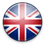## Rotating an image on a web pageNovember 21, 2009

This post is about rotating an image on a web page – that is not rotating more images (slide-showing them). It’s about rotating an image with an angle. Why would that be useful? If you want to put an image rotated by an angle on a web page, the easiest would be to make a new image, with the original image rotated.

Well, the need appeared when I started to build a Mac OS Dashboard Widget, that had to show some values on an analog display. I needed to rotate the image with the needle by an angle proportional to the value. The angle of rotation had to be modifiable from JavaScript. The Dashboard engine is based on WebKit component, and the widget itself is able to run on Safari as well. So the need to rotate an image on a web page is the generic issue that I had to solve.

The solution is to use the <canvas> tag, available in HTML 5 and  implemented by Safari browser, Gecko-based browsers (like Firefox) and Opera. The image would be drawn on the canvas that can rotate.

The issue with this method is that the canvas rotates around the top left corner. The needle has to be rotated around its rotation center, which is located somewhere inside the image, not on its corner. So a trigonometric transformation had to be implemented (which includes translation and rotation), so that the needle will appear to rotate around its rotation center, not the image corner.

The JavaScript code that implements the rotation is the following:

```
function drawImg(canvas, src, angle, centru_ox_delta, centru_oy_delta, canvas_width) {
var myImg = new Image();
myImg.src = src;
myImg.angle = angle;
myImg.canvas = canvas;
myImg.oxd = centru_ox_delta;
myImg.oyd = centru_oy_delta;
myImg.canvas_w = canvas_width;
}

var canvas = document.getElementById(this.canvas);
if(canvas)
var ctx = document.getElementById(this.canvas).getContext('2d');
if (ctx) {
ctx.save();
ctx.clearRect(0,0,250,250);
ctx.translate( this.canvas_w*Math.sqrt(2)/2*(0.7 - Math.cos((this.angle+45) * Math.PI / 180)), this.canvas_w*Math.sqrt(2)/2*(0.7 - Math.sin((this.angle+45) * Math.PI / 180)) );
ctx.rotate(this.angle * Math.PI / 180);
ctx.drawImage(this, this.canvas_w/2-this.oxd, this.canvas_w/2-this.oyd);
ctx.restore();
};
}

```

The “drawImg” function creates a new Image object, sets its source (the URL of the needle image), sets its “loaded” callback and sets some other custom properties of the object, that will be used in the callback functon. The callback function is “imageLoadedRotate”, which obtains the 2d context of the canvas, with which we can draw images on the canvas. We first position the context to the new position, we rotate it, and then we draw the image. The image will appear rotated because the context is rotated. The context properties are first saved, so we can restore them afterwards, because the context will be reused and we need to rotate the images relative to their initial position (vertical, in our case).

The “imageLoadedRotate” function also makes the transformation between the degrees value that we use and the radians value that the rotation function understands.

The parameters of the “drawImg” function are:

• canvas – the id of the canvas to use (<canvas id=”canvas_id” width=”250″ height=”250″></canvas>)
• src – the URL of the image to draw
• angle – the angle of rotation
• centru_ox_delta – the Ox coordinate of the rotation center of the image, relative to the image’s left edge
• centru_oy_delta – the Oy coordinate of the rotation center of the image, relative to the image’s top edge
• canvas_width – the canvas size (width=height, because we used only square canvases, for ease of calculation*